Refer to our Texas Go Math Grade 1 Answer Key Pdf to score good marks in the exams. Test yourself by practicing the problems from Texas Go Math Grade 1 Lesson 4.1 Answer Key Use Pictures to Add To.

Essential Question
How con you use pictures to show adding to, or joining?
Explanation:
By counting the pictures we can add the pictures.

Explore
Draw to show adding to, or joining Write how many there are.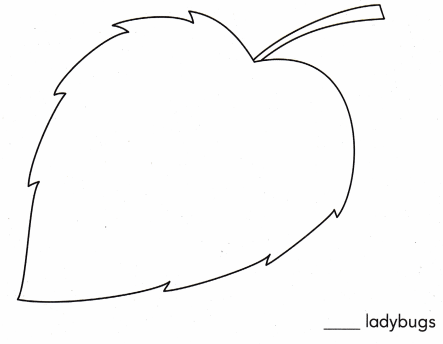Explanation: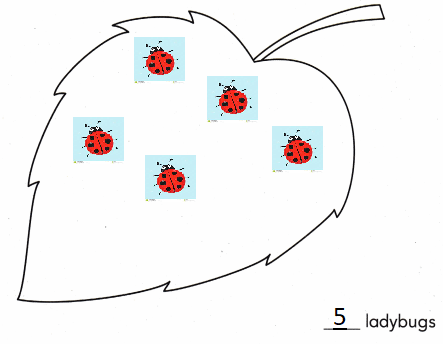3 + 3 = 6 lady bugs

For The Teacher

• Read the following problem. Have children draw a picture to show the problem. There are 3 ladybugs on a leaf. 2 more ladybugs join them. How many ladybugs are there?

Math Talk
Mathematical Processes
How does your drawing show the problem? Explain.

Model and Draw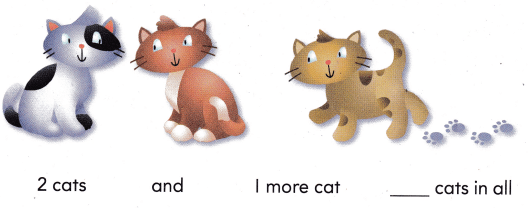Explanation:there are 2 + 1 = 3 cats in all

Share and Show

Write how many.

Question 1.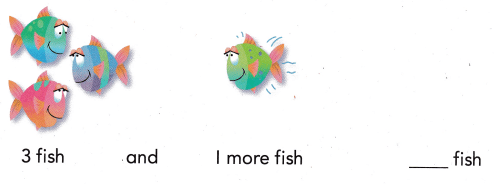Explanation:
There are 3 + 1 = 5 fishes in all

Question 2.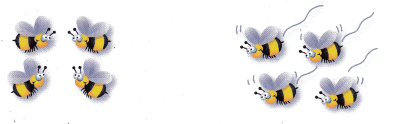There are 4 bees. 4 more bees join them. How many bees are there?
____ bees
8 bees
Explanation:
there are 4 + 4 = 8 bees in all

Problem Solving

Write how many.

Question 3.Mike sees 2 butterflies.
Then 4 more butterflies join them.
How many butterflies are there?
____ butterflies
2 + 4 = 6
Explanation:
6 butterflies
there are 2 + 4 = 6 butterflies in all

Write or draw to solve.

Question 4.
There are 4 ants. 5 more ants join them. How many ants are there?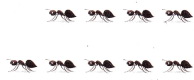_____ ants
9 Ants
Explanation:
the sum of 4 and 5 is 9

Question 5.
H.O.T. Evan and Luke see 8 worms on the path. Luke sees 2 more worms than Evan. Evan sees 3 worms. How many worms does Luke see?Explanation:
8 + 2 + 3 = 13
13 worms that Luke see

Question 6.
H.O.T. Multi-Step There are 3 red birds. 2 blue birds join them. Then 1 more red bird joins them. How many birds are there?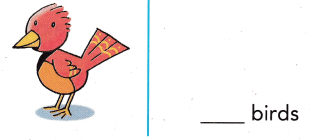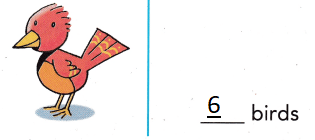Explanation:
3 + 2 + 1 = 6
6 birds are there in all

Question 7.
Mr. Tilly feeds a seal. He gives the seal 3 fish. Then he gives the seal 3 more fish. How many fish did Mr. Tilly give the seal?(A) 4
(B) 6
(C) 7
Explanation:
3 + 3 = 6
6 fish that Mr. Tilly give the seal

Question 8.
A penguin eats some fish. He eats 4 small fish and 3 big fish. How many fish does the penguin eat?(A) 7
(B) 1
(C) 6
Explanation:
4 + 3 = 7
7 fish that the penguin eat

Question 9.
Representations There are 4 ducks in the pond. 2 more ducks join them. How many ducks are ¡n the pond now?
(A) 2
(B) 6
(C) 4
Explanation:
4 + 2 = 6
6 ducks are ¡n the pond now

Question 10.
Gavin sees 2 turtles on a log. Then he sees 6 more turtles join them. How many turtles are on the log now?
(A) 6
(B) 8
(C) 2
Explanation:
2 + 6 = 8
8 turtles are on the log now

Question 11.
Texas Test Prep How many bees are there?(A) 3
(B) 8
(C) 7
Explanation:
2 + 5 = 7

Take Home Activity

• Have your child use stuffed animals or other toys to show 3 animals. Then odd to the group showing 2 more animals. Ask how many animals there are, Repeat for other combinations of animals with totals up to 10.

### Texas Go Math Grade 1 Lesson 4.1 Homework and Practice Answer Key

Write how many.

Question 1.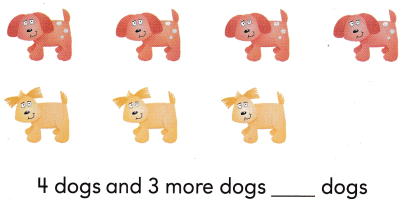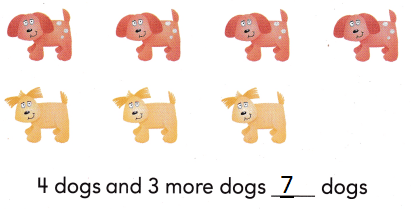Explanation:
4 + 3 = 7
there are 7 dogs in all

Problem Solving

Write or draw to solve.

Question 2.
4 oranges fall off the tree. Then 2 more oranges fall off. How many oranges fall off the tree?
____ oranges
6 oranges
Explanation:
4 + 2 = 6
6 oranges fall off the tree

Question 3.
Ava and Emma eat 5 strawberries. Emma eats 1 more than Ava. Ava eats 2 strawberries. How many strawberries does Emma eat?
___ strawberries
3 strawberries
Explanation:
2 + 1 = 3
3 strawberries that Emma eat

Texas Test Prep

Lesson Check

Question 4.
Ethan watches a baseball game. One team scores 2 runs. Then the same team scores 6 more runs. How many runs does the team score?
(A) 9
(B) 4
(C) 8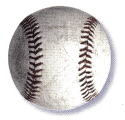Explanation:
6 + 2 = 8
8 runs that the team score

Question 5.
There are 6 friends playing kickball. 3 more friends join them. How many friends are playing kickball?
(A) 8
(B) 3
(C) 9
Explanation:
6 + 3 = 9
9 friends are playing kickball

Question 6.
How many soccer balls are there? 3 soccer balls and 5 more soccer balls.
(A) 2 soccer balls
(B) 8 soccer balls
(C) 7 soccer balls
Explanation:
3 + 5  = 8
8 soccer balls.

Question 7.
Multi-Step Matt is playing with 4 friends. 5 friends join them. Then 3 more friends join them. How many friends is Matt playing with?
(A) 12
(B) 8
(C) 13